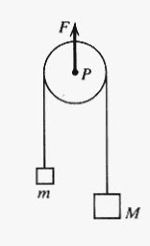# Easy problem about a pulley accelerating

LCSphysicist
Homework Statement:
Summarizing, it is a system with a pulley being pulled by a force F, with two masses hanging.
Relevant Equations:
F = ma
Xm + XM = 2XpThat is the classic problem involving pulley and masses, I can find everything that is requested, but there is a big problem, i don't know how to justify that the tensions in both side are equal to half of the force.

Mentor
Is the pulley accelerating?

LCSphysicist
Is the pulley accelerating?
Yes, the pulley is accelerating, which if it is assumed that T = F/2 (i don't know why), we can know the acceleration is:
(M+m)F/4Mm - g

Staff Emeritus
Homework Helper
Gold Member
Yes, the pulley is accelerating, which if it is assumed that T = F/2 (i don't know why), we can know the acceleration is:
(M+m)F/4Mm - g
What is the mass of the pulley?

Homework Statement:: Summarizing, it is a system with a pulley being pulled by a force F, with two masses hanging.
Relevant Equations:: F = ma
Xm + XM = 2Xp

i don't know how to justify that the tensions in both side are equal to half of the force.
why do not you write the 2 Newton law for each body

Homework Helper
Gold Member
..., I can find everything that is requested, but there is a big problem, i don't know how to justify that the tensions in both side are equal to half of the force.
Unwrap the string from around the pulley and place it, together with the weights on an imaginary horizontal flat surface.
Pull the big weight with a horizontal force of magnitude Mg.
Simultaneously, pull the small weight with a horizontal force of magnitude mg, but in the opposite direction.

There is a resultant force acting on the whole system (M+m), which has certain acceleration.

In between those weights, you will have the string, which will be loaded with a tension of magnitude Mg-mg, which equals (M+m)a, being a the acceleration of the system as a whole.

Because of the above, the pulley should be rotating (clockwise) and the distance between M and the pulley should be increasing.

What does the work to make the mass-less pulley rotate is not any difference in right-left tension, but the resulting force acting on the system.
As your imaginary flat arrangement moves horizontally, just let the edge of the stationary pulley contact the sliding horizontal string, and, if friction is present, it will start rotating.

Last edited:
Mentor
Yes, the pulley is accelerating, which if it is assumed that T = F/2 (i don't know why),
Is the pulley assumed to be massless? If so, what will Newton's 2nd law tell you?